Want to share your content on R-bloggers? click here if you have a blog, or here if you don't.

No need to do politics. Just take a statistical course. And I do not talk about misinterpretation of statistics, but I talk about the mathematical foundations of statistical tests.
Consider the following parametric test, with a one-dimensional parameter: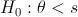versus, for some (fixed)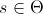. A standard way of doing such a test is to consider an rejection region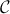. The test works as follows: consider a sample,
• if, then we accept• if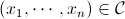, the we rejectFor instance, consider the case of a Bernoulli sample, with probability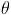. The standard idea is to defineThe rejection region is then based on statistic,
• if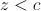, then we accept• if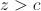, the we rejectwhere thresholdis taken so that the probability to make a first type error is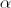(say 5%) using the Gaussian approximation for z. Here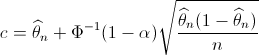Thus, the acceptation region is then the green area below, while the rejection region is the red one, for.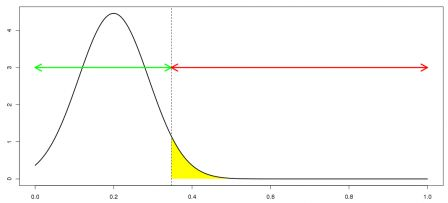Consider now the exact opposite test (with the same),versus. Here, we use the same statistics, and the test is
• if, then we accept• if, the we rejectwhere nowThus, now, the acceptation region is then the green area below, while the rejection region is the red one.So if we summarize what we just said,
• in the region on the left below, both test agree that• in the region on the right below, both test agree that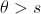• and in the region in blue, in the middle, the two tests disagree (one claims that, and the other one that)Here is the evolution of the region as a function of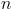(the size of the sample) when the sample frequency is 20%. With a small sample size, we can hardly say anything.
n=seq(1,100)
p=0.2
x1=p+qnorm(.95)*sqrt(p*(1-p)/n)
x2=p+qnorm(.05)*sqrt(p*(1-p)/n)
plot(n,x1,type="l",ylim=c(0,1))
polygon(c(n,rev(n)),c(x1,rev(x2)),col="light blue",border=NA)
lines(n,x1,lwd=2,col="red")
lines(n,x2,lwd=2,col="red")One might say that those bounds are based on a Gaussian approximation which is not correct whenis too small. So we can compute exact bounds,
y1=qbinom(.95,size=n,prob=p)/n
y2=qbinom(.05,size=n,prob=p)/n
polygon(c(n,rev(n)),c(y1,rev(y2)),col="blue",border=NA)
lines(n,y1,lwd=2,col="red")
lines(n,y2,lwd=2,col="red")
and we get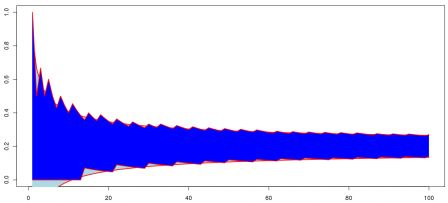This is what we can observe if we use R statistical procedures, either the asymptotic one,
> prop.test(2,10,.5,alternative="less")

1-sample proportions test with continuity correction

data:  2 out of 10, null probability 0.5
X-squared = 2.5, df = 1, p-value = 0.05692
alternative hypothesis: true p is less than 0.5
95 percent confidence interval:
0.0000000 0.5100219
sample estimates:
p
0.2

> prop.test(2,10,.5,alternative="greater")

1-sample proportions test with continuity correction

data:  2 out of 10, null probability 0.5
X-squared = 2.5, df = 1, p-value = 0.943
alternative hypothesis: true p is greater than 0.5
95 percent confidence interval:
0.04368507 1.00000000
sample estimates:
p
0.2
or a more accurate one
> binom.test(2,10,.5,alternative="less")

Exact binomial test

data:  2 and 10
number of successes = 2, number of trials = 10, p-value = 0.05469
alternative hypothesis: true probability of success is less than 0.5
95 percent confidence interval:
0.0000000 0.5069013
sample estimates:
probability of success
0.2

> binom.test(2,10,.5,alternative="greater")

Exact binomial test

data:  2 and 10
number of successes = 2, number of trials = 10, p-value = 0.9893
alternative hypothesis: true probability of success is greater than 0.5
95 percent confidence interval:
0.03677144 1.00000000
sample estimates:
probability of success
0.2Here, when the sample frequency is 20% andis equal to 10, we accept at the same time that theta is higher than 50% and lower than 50%.
And obviously it is not only a theoretical problem: it has obviously some strong implications. This morning, a good friend mentioned a post published some months ago, online here, about discrimination, and the lack of women with academic positions in mathematics, in France. As claimed by the author of the postA Paris VI, meilleure université française selon son président, sur 11 postes de maitres de conférences, 5 filles classées premières. Il y a donc des filles excellentes ? A Toulouse, sur 4 postes, 2 filles premières. Parité parfaite. Mais à côté de cela, Bordeaux, 4 postes, 0 fille première. Littoral, 3 postes, 0 fille, Nice, 5 postes, 0 fille, Rennes, 7 postes, 0 fille…“.
Consider the latter one: in Rennes, out of 7 people hired last year, no woman. So in some sense, it looks obvious that there is some kind of discrimination ! Zero out of seven ! Well, if we consider the fact that around 30% of PhD thesis in mathematics were defended by women those years, we can also try to see is there if no “positive discrimination“, i.e. test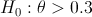where theta is the probability to hire a woman (just to be a little bit provocative).
> prop.test(0,7,.3,alternative="less")

1-sample proportions test with continuity correction

data:  0 out of 7, null probability 0.3
X-squared = 1.7415, df = 1, p-value = 0.09347
alternative hypothesis: true p is less than 0.3
95 percent confidence interval:
0.0000000 0.3719021
sample estimates:
p
0

Warning message:
In prop.test(0, 7, 0.3, alternative = "less") :
Chi-squared approximation may be incorrect
> binom.test(0,7,.3,alternative="less")

Exact binomial test

data:  0 and 7
number of successes = 0, number of trials = 7, p-value = 0.08235
alternative hypothesis: true probability of success is less than 0.3
95 percent confidence interval:
0.0000000 0.3481637
sample estimates:
probability of success
0
With no woman hired that year, we can still pretend that there was some kind of “positive discrimination“. An note that we do accept – with more confidence – the assumption of “positive discrimination” if we look at all universities together,
> prop.test(5+2,11+4+4+3+5+7,.3,alternative="less")

1-sample proportions test with continuity correction

data:  5 + 2 out of 11 + 4 + 4 + 3 + 5 + 7, null probability 0.3
X-squared = 1.021, df = 1, p-value = 0.1561
alternative hypothesis: true p is less than 0.3
95 percent confidence interval:
0.0000000 0.3556254
sample estimates:
p
0.2058824

> binom.test(5+2,11+4+4+3+5+7,.3,alternative="less")

Exact binomial test

data:  5 + 2 and 11 + 4 + 4 + 3 + 5 + 7
number of successes = 7, number of trials = 34, p-value = 0.1558
alternative hypothesis: true probability of success is less than 0.3
95 percent confidence interval:
0.0000000 0.3521612
sample estimates:
probability of success
0.2058824
So obviously, with small sample, almost anything can be claimed !